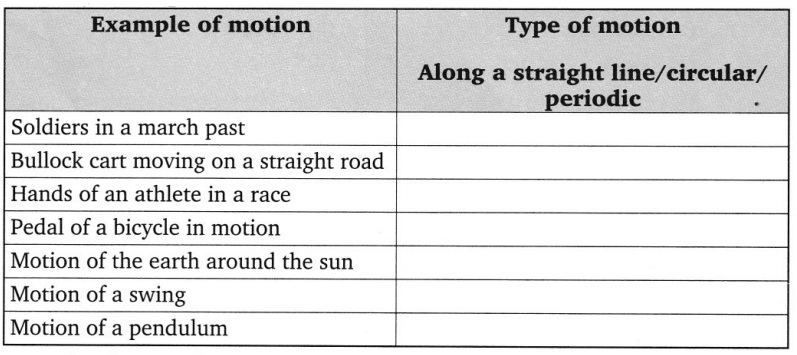# Grade 7 Motion and Time Worksheets

A. Answer the following questions in short:

1. Classify the following as motion along a straight line, circular or oscillatory motion:
(i) Motion of your hands while running.
(ii) Motion of a horse pulling a cart on a straight road.
(iii) Motion of a child in a merry-go-round.
(iv) Motion of a child on a see-saw.
(v) Motion of the hammer of an electric bell.
(vi) Motion of a train on a straight bridge.
2. A simple pendulum takes 32 seconds to complete 20 oscillations. What is the time period of the pendulum?
3. The distance between two stations is 240 km. A train takes 4 hours to cover this distance. Calculate the speed of the train.
4. The odometer of a car reads 57321.0 km when the clock shows the time 08:30 AM. What is the distance moved by the car, if at 08:50 AM, the odometer reading has changed to 57336.0 km? Calculate the speed of the car in km/min during this time. Express the speed in km/h also.
5. Salma takes 15 minutes from her house to reach her school on a bicycle. If the bicycle has a speed of 2 m/s, calculate the distance between her house and the school.
6. Show the shape of the distance-time graph for the motion in the following cases:
(i) A car moving with a constant speed.
(ii) A car parked on a side road.7. Suppose the two photographs, shown in fig. 13.1 and fig. 13.2 (in NCERT textbook) had been taken at an interval of 10 seconds. If a distance of 100 metres is shown by 1 cm in these photographs, calculate the speed of the blue car.
8. Adjoining figure shows the distancetime graph for the motion of two vehicles A and B. Which one of them is moving faster?9. Why are standard units used in measurements?
10. How time was measured when pendulum clocks were not available?
11. Why do you think accurate measurements of time became possible much after accurate measurement of length and mass?

B. Which of the following are not correct?

(i) The basic unit of time is second.
(ii) Every object moves with a constant speed.
(iii) Distances between two cities are measured in kilometres.
(iv) The time period of a given pendulum is not constant.
(v) The speed of a train is expressed in m/h.

C. Tick (✓) the correct option:

1. Which of the following relations is correct ?
(a) Speed = Distance × Time
(b) Speed =$\frac{\text { Distance }}{\text { Time }}$
(c) km/h =$\frac{\text { Time }}{\text { Distance }}$
(d) m/s =$\frac{1}{\text { Distance } \times \text { Time }}$

2. The basic unit of speed is:
(a) km/min
(b) m/min
(c) km/h
(d) m/s

3. A car moves with a speed of 40 km/h for 15 minutes and then with a speed of 60 km/h for the next 15 minutes. The total distance covered by the car is:
(a) 100 km
(b) 25 km
(c) 15 km
(d) 10 km

4. Which of the following distance-time graphs shows a truck moving with speed which is not constant?D. Fill in the blanks:

1. Speed is a ………………. quantity.
2. The distance covered by a vehicle is measured by ………………. .
3. A device which measures time by the flow of sand is ……………… .
4. If the distance-time graph is a curved line then its speed is ………………… .

E. Match the following:

 ‘A’ ‘B’ 1. Periodic motion a. Twenty-four hours 2. Time b. Non-uniform speed 3. Pendulum c. Gap between two events 4. Solar day d. Movement of earth on its axis 5. Zig-zag graph e. A heavy mass suspended from string

F. Identify the type of motion in each case:G. Speeds of some living organisms are given in the table given below. You can calculate the speeds in m/s yourself: